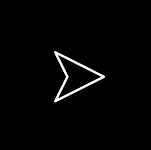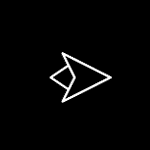# 5.2加速度

## 5.2.1一个轴上的加速度

package
{
import flash.display.Sprite;
import flash.events.Event;

public class Acceleration1 extends Sprite
{
private var ball:Ball;
private var vx:Number = 0;
private var ax:Number = .2;

public function Acceleration1()
{
init();
}

private function init():void
{
ball = new Ball();
ball.x = 50;
ball.y = 100;
}
private function onEnterFrame(event:Event):void
{
vx += ax;
ball.x += vx;
}
}
}

package
{
import flash.display.Sprite;
import flash.events.Event;
import flash.events.KeyboardEvent;
import flash.ui.Keyboard;

public class Acceleration2 extends Sprite
{
private var ball:Ball;
private var vx:Number = 0;
private var ax:Number = 0;
public function Acceleration2()
{
init();
}

private function init():void
{
ball = new Ball();
ball.x = stage.stageWidth / 2;
ball.y = stage.stageHeight / 2;
}

private function onKeyDownHandler(event:KeyboardEvent):void
{
if (event.keyCode == Keyboard.LEFT)
{
ax = -0.2;
}
else if (event.keyCode == Keyboard.RIGHT)
{
ax = 0.2;
}
}

private function onKeyUpHandler(event:KeyboardEvent):void
{
ax = 0;
}

private function onEnterFrame(event:Event):void
{
vx +=  ax;
ball.x +=  vx;
}
}
}

## 5.2.2两个轴上的加速度

package
{
import flash.display.Sprite;
import flash.events.Event;
import flash.events.KeyboardEvent;
import flash.ui.Keyboard;

public class Acceleration3 extends Sprite
{
private var ball:Ball;
private var vx:Number = 0;
private var vy:Number = 0;
private var ax:Number = 0
private var ay:Number = 0

public function Acceleration3()
{
init();
}

private function init():void
{
ball = new Ball();
ball.x = stage.stageWidth / 2;
ball.y = stage.stageHeight / 2;
}

private function onKeyDownHandler(event:KeyboardEvent):void
{
switch(event.keyCode)
{
case Keyboard.LEFT :
ax = -0.2;
break;

case Keyboard.RIGHT :
ax = 0.2;
break;

case Keyboard.UP :
ay = -0.2;
break;

case Keyboard.DOWN :
ay = 0.2;
break;

default :
break;
}
}

private function onKeyUpHandler(event:KeyboardEvent):void
{
ax = 0;
ay = 0;
}

private function onEnterFrame(event:Event):void
{
vx += ax;
vy += ay;
ball.x += vx;
ball.y += vy;
}
}
}

## 5.2.3重力加速度

private var gravity:Number = 0.1;

private function onEnterFrame(event:Event):void
{
vx += ax;
vy += ay;
vy += gravity;
ball.x += vx;
ball.y += vy;
}

## 5.2.4角加速度

var force:Number = 10;
var angle:Number = 45; // degrees. Need to convert!
var ax:Number = Math.cos(angle * Math.PI / 180) * force;
var ay:Number = Math.sin(angle * Math.PI / 180) * force;

package
{
import flash.display.Sprite;
import flash.events.Event;

public class FollowMouse2 extends Sprite
{
private var arrow:Arrow;
private var vx:Number = 0;
private var vy:Number = 0;
private var force:Number = 0.5;

public function FollowMouse2()
{
init();
}

private function init():void
{
arrow = new Arrow();
}
private function onEnterFrame(event:Event):void
{
var dx:Number = mouseX - arrow.x;
var dy:Number = mouseY - arrow.y;
var angle:Number = Math.atan2(dy, dx);
arrow.rotation = angle * 180 / Math.PI;
var ax:Number = Math.cos(angle) * force;
var ay:Number = Math.sin(angle) * force;
vx += ax;
vy += ay;
arrow.x += vx;
arrow.y += vy;
}
}
}

OK，让我们齐心协力，看看到底能够走多远吧。

## 5.2.5制作飞船

package
{
import flash.display.Sprite;

public class Ship extends Sprite
{
public function Ship()
{
draw(false);
}

public function draw(showFlame:Boolean):void
{
graphics.clear();
graphics.lineStyle(1, 0xffffff);
graphics.moveTo(10, 0);
graphics.lineTo(-10, 10);
graphics.lineTo(-5, 0);
graphics.lineTo(-10, -10);
graphics.lineTo(10, 0);

if(showFlame)
{
graphics.moveTo(-7.5, -5)
graphics.lineTo(-15, 0);
graphics.lineTo(-7.5, 5);
}
}
}
}### 飞船控制

OK，飞船已经有了，接下来就要控制它了。刚才说过，要执行三种控制：左转，右转和点火，分别由左右上三个键控制。本例的代码与 Acceleration3.as 非常相似，用到事件处理函数 keyDown 和 keyUp 还有一个 switch 语句对按键进行分类处理。 先把所有代码给大家，随后再进行解释(ShipSim.as)：

package
{
import flash.display.Sprite;
import flash.events.Event;
import flash.events.KeyboardEvent;
import flash.ui.Keyboard;

public class ShipSim extends Sprite
{
private var ship:Ship;
private var vr:Number = 0;
private var thrust:Number = 0;
private var vx:Number = 0;
private var vy:Number = 0;
public function ShipSim()
{
init();
}

private function init():void
{
ship = new Ship();
ship.x = stage.stageWidth / 2;
ship.y = stage.stageHeight / 2;
ship.draw(true);
}

private function onKeyDownHandler(event:KeyboardEvent):void
{
switch(event.keyCode)
{
case Keyboard.LEFT :
vr = -5;
break;

case Keyboard.RIGHT :
vr = 5;
break;

case Keyboard.UP :
thrust = 0.2;
ship.draw(true);
break;

default :
break;
}
}

private function onKeyUpHandler(event:KeyboardEvent):void
{
vr = 0;
thrust = 0;
ship.draw(false);
}

private function onEnterFrame(event:Event):void
{
ship.rotation += vr;
var angle:Number = ship.rotation * Math.PI / 180;
var ax:Number = Math.cos(angle) * thrust;
var ay:Number = Math.sin(angle) * thrust;
vx += ax;
vy += ay;
ship.x += vx;
ship.y += vy;
}
}
}

private var vr:Number = 0;

case Keyboard.LEFT :
vr = -5;
break;
case Keyboard.RIGHT :
vr = 5;
break;

private var thrust:Number = 0;

case Keyboard.UP :
thrust = 0.2;
ship.draw(true);
break;

private function onKeyUpHandler(event:KeyboardEvent):void
{
vr=0;
thrust=0;
ship.draw(false);
}

private function onEnterFrame(event:Event):void
{
ship.rotation += vr;
var angle:Number = ship.rotation * Math.PI / 180;
var ax:Number = Math.cos(angle) * thrust;
var ay:Number = Math.sin(angle) * thrust;
vx += ax;
vy += ay;
ship.x += vx;
ship.y += vy;
}

2006 - 2023，推荐分辨率1024*768以上，推荐浏览器Chrome、Edge等现代浏览器，截止2021年12月5日的访问次数：1872万9823 站长邮箱